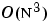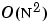MATLAB Function Referencecholupdate

Rank 1 update to Cholesky factorization

Syntax

• ```R1 = cholupdate(R,x)
R1 = cholupdate(R,x,'+')
R1 = cholupdate(R,x,'-')
[R1,p] = cholupdate(R,x,'-')
```

Description

```R1 = cholupdate(R,x) ``` where `R = chol(A)` is the original Cholesky factorization of `A`, returns the upper triangular Cholesky factor of `A + x*x'`, where `x` is a column vector of appropriate length. `cholupdate `uses only the diagonal and upper triangle of `R`. The lower triangle of `R` is ignored.

```R1 = cholupdate(R,x,'+') ``` is the same as `R1 = cholupdate(R,x)`.

```R1 = cholupdate(R,x,'-') ``` returns the Cholesky factor of `A - x*x'`. An error message reports when R is not a valid Cholesky factor or when the downdated matrix is not positive definite and so does not have a Cholesky factorization.

```[R1,p] = cholupdate(R,x,'-') ``` will not return an error message. If `p` is `0`, `R1` is the Cholesky factor of `A - x*x`'. If `p` is greater than `0`, `R1` is the Cholesky factor of the original `A`. If `p` is `1`, `cholupdate` failed because the downdated matrix is not positive definite. If `p` is `2`, `cholupdate` failed because the upper triangle of `R` was not a valid Cholesky factor.

Remarks

`cholupdate `works only for full matrices.

Example

• ```A = pascal(4)
A =

1     1     1     1
1     2     3     4
1     3     6    10
1     4    10    20

R = chol(A)
R =

1     1     1     1
0     1     2     3
0     0     1     3
0     0     0     1
x = [0 0 0 1]';
```

This is called a rank one update to `A` since `rank(x*x')` is `1`:

• ```A + x*x'
ans =
```

• ```     1     1     1     1
1     2     3     4
1     3     6    10
1     4    10    21
```

Instead of computing the Cholesky factor with `R1 = chol(A + x*x')`, we can use `cholupdate`:

• ```R1 = cholupdate(R,x)
R1 =
```

• ```    1.0000    1.0000    1.0000    1.0000
0    1.0000    2.0000    3.0000
0         0    1.0000    3.0000
0         0         0    1.4142
```

Next destroy the positive definiteness (and actually make the matrix singular) by subtracting `1` from the last element of `A`. The downdated matrix is:

• ```A - x*x'
ans =

1     1     1     1
1     2     3     4
1     3     6    10
1     4    10    19
```

Compare `chol` with `cholupdate`:

• ```R1 = chol(A-x*x')
??? Error using ==> chol
Matrix must be positive definite.
R1 = cholupdate(R,x,'-')
??? Error using ==> cholupdate
Downdated matrix must be positive definite.
```

However, subtracting `0.5` from the last element of `A` produces a positive definite matrix, and we can use `cholupdate` to compute its Cholesky factor:

• ```x = [0 0 0 1/sqrt(2)]';
R1 = cholupdate(R,x,'-')
R1 =
1.0000    1.0000    1.0000    1.0000
0    1.0000    2.0000    3.0000
0         0    1.0000    3.0000
0         0         0    0.7071
```

Algorithm

`cholupdate` uses the algorithms from the LINPACK subroutines `ZCHUD` and `ZCHDD`. `cholupdate` is useful since computing the new Cholesky factor from scratch is analgorithm, while simply updating the existing factor in this way is analgorithm.

See Also

`chol`, `qrupdate`

References

  Dongarra, J.J., J.R. Bunch, C.B. Moler, and G.W. Stewart, LINPACK Users' Guide, SIAM, Philadelphia, 1979.

© 1994-2005 The MathWorks, Inc.